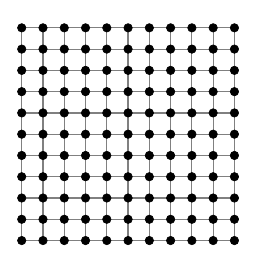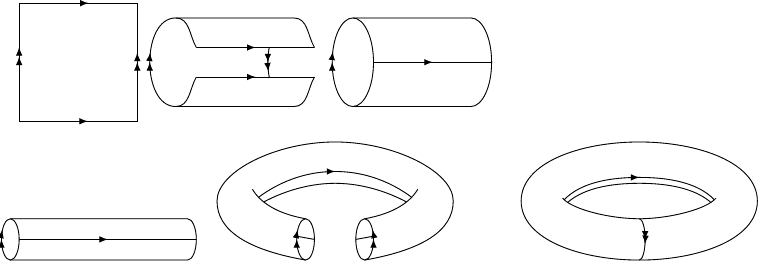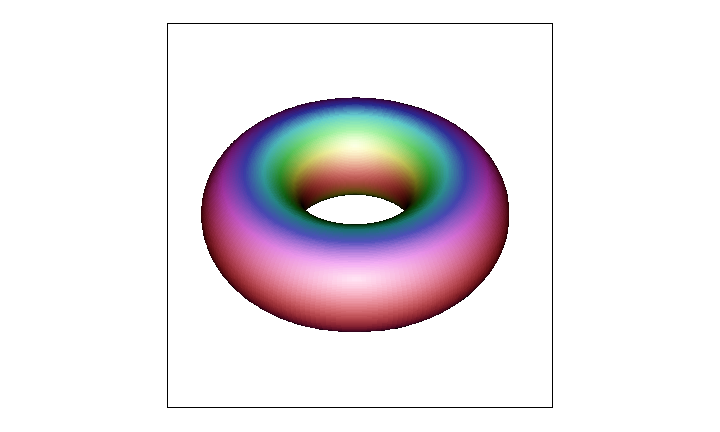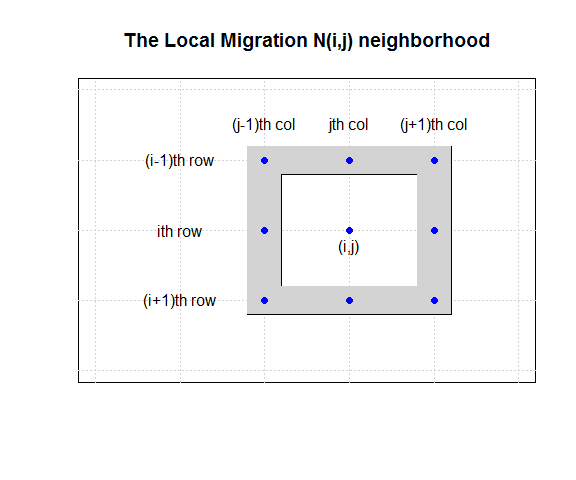August 7, 2015

## Introduction

• This is a computational biology project developed as a student research project sponsored by the MSEIP Grant between BMCC and City Tech, CUNY.

• It aims at creating a computer simulation and animation of the spatial interactions between two kinds of species living on a torus.

• The simulation illustrates the effects of spatial structure on population dynamics and the emergence of complex spatial wave patterns.

• The habitat for spatial interactions is modeled by a 2D lattice with periodic boundary conditions, which wrap the rectangular grid into a torus.

## The Classical Nicholson-Bailey Model

• In the 1930s Nicholson and Bailey proposed a discrete-time model for the population dynamics of insect hosts and their parasitoids.

• Parasitoids resemble parasites as they lay eggs and grow inside a host, but also resemble predators in that they eventually kill their host.

• A key characteristic of the classical Nicholson-Bailey model is that both populations undergo unstable oscillations until first the host and then the parasitoid population dies out.

• This project investigates the population dynamics of an extended Nicholson-Bailey model, having spatial distribution of both host and parasitoid that can be used to investigate the effect of spatial structure on stabilizing the population dynamics of the species.

## A Spatial Model of Interacting Species

The extended Nicholson-Bailey model incorporates spatial structure, by simulating the dynamics of spatial interactions between two species living on a 2D lattice with periodic boundary conditions. We have two dynamics in play:

• A population dynamics modeled by the classical Nicholson-Bailey two-parameter family of models for coupled interactions between species, extended to incorporate space.

• A migration dynamics, modeled by the weighted average of the current population density and the average inflow of migrating species from the nearest 8-neighbor migration zone.

## A Spatial Model of Interacting Species

• In our spatially extended model, hosts and parasitoids can move from the location in which they were born to any one of the nearest eight neighboring cells in a 2D grid.

• For the purposes of dispersal, the universe is assumed to have wrap-around boundaries for both species in a way that creates a torus-shaped universe.

• The interaction is capable of producing beautiful spatial wave patterns that fluctuate with host and parasitoid abundance.

## Periodic Boundary Conditions on the 2D Grid

The model imposes periodic boundary conditions on the 2D grid, which glue the opposite sides of the grid into a torus.On the Rectangular 2D grid:

• we add an extra layer to the grid to represent the periodic boundary conditions imposed on the model.
• each cell in the grid interacts with the 8 nearest neighbors only.

## Wrapping the 2D Lattice into a Torus

Periodic Boundary Rules:

• the right neighbor of a point in the far right column is a point in the far left column in the same row.
• the bottom neighbor of a point in the bottom row is a point in the top row in the same column.## A Torus-shaped Universe Hosting the Species## The Classical Nicholson-Bailey Model

• The Nicholson-Bailey model is a 2D coupled system of difference equations for the population dynamics of hosts and parasitoids living in a 1D world:

$H_{t+1} = H_t e^{r-aP_t}$ $P_{t+1} = H_t (1-e^{-aP_t})$

• Space matters, however, because the two populations tend to interact and breed locally and might not migrate over long distances relative to the range of the species but would certainly migrate locally.

• Some spatial models are analytically tractable but most are too complex to get any analytical results and using computer simulations is the only means of understanding these models.

## The Spatial Nicholson-Bailey Model

• We incorporate space using a 2D grid of size $$200\times 200$$; for each cell $$(i,j)$$ in the grid the dynamics are given by the classical Nicholson-Bailey model, and we also allow that hosts and parasitoids disperse to all 8 nearest neighbors with given migration rates $$d_h$$ and $$d_p$$:

$H_{i,j}(t+1)=H^{*}_{i,j}(t)e^{r-aP^{*}_{i,j}(t)}$ $P_{i,j}(t+1)=H^{*}_{i,j}(t)(1-e^{-aP^{*}_{i,j}(t)})$

• Nicholson-Bailey population parameters $$r=0.4$$ and $$a=0.1$$.

## 2D Grid for the Migration Dynamics

$$z_{i,j}(t)$$ is the time $$t$$ population size in cell $$(i,j)$$ of the grid. The neighborhood $$N(i,j)$$ of cell $$(i,j)$$ for the 8 nearest neighbors.## Migration Through Local Averaging

The migration dynamics over time is given by repeatedly applying a local averaging. The update process at each time step and for each cell $$(i,j)$$ on the grid is given by the weighted average:

$z_{i,j}(t+1)=(1-w)z_{i,j}(t)+w\,z^{*}_{i,j}(t),$

where the weight $$w<1$$ is the migration rate and it sets the proportion of the new $$z$$ value that results from the local average, with the remainder $$1-w$$ derived from the current $$z$$ value in the cell.

The average flow from the 8 nearest neighbors is given by

$z^{*}_{i,j}(t)=\frac{1}{8}\sum_{(m,n)\in N(i,j)}z_{m,n}(t)$

## Migration Dynamics for the Species

Mathematically, the spatial model for an arbitrary cell $$(i,j)$$ defining the migration dynamics for the two populations at time $$t$$ is given by:

$H^{*}_{i,j}(t+1)=(1-d_h)H_{i,j}(t)+\frac{d_h}{8}\sum_{(m,n)\in N(i,j)}H_{m,n}(t)$ $P^{*}_{i,j}(t+1)=(1-d_p)P_{i,j}(t)+\frac{d_p}{8}\sum_{(m,n)\in N(i,j)}P_{m,n}(t),$

where $$N(i,j)$$ is the nearest neighborhood around cell $$(i,j)$$.

• The migration rates for host and parasites are $$d_h=0.1$$ and $$d_p=0.9$$ for relatively sedentary hosts and highly mobile parasitoids.

## Programming with R

• We coded the simulations using the high-level programming language R, which allows for very compact code that can be quickly developed by using a mixture of functional and procedural programming.

• This programming approach allows the entire model dynamics to be coded in less than 50 lines of code, making it ideal for quick development and for implementing student projects.

• We bundled the simulation into an animation that can be embedded into a pdf or html document, featuring the time evolution of the spatial interactions between the species, with given periodic boundary conditions.

## Simulating and Animating the Spatial Dynamics

• We created an animation featuring the time evolution of the spatial interactions between the species living on a torus, revealing beautiful spatial wave patterns produced by the interfering waves of the population density.

• The spatial waves are visualizing the fluctuating in time abundance of the species living in their torus-shaped universe.

Simulation Parameters:

• Nicholson-Bailey population parameters: $$r=0.4$$ and $$a=0.1$$.
• Migration rates: $$d_h=0.1$$ and $$d_p=0.9$$.
• At $$t=0$$ we start with 500 host population and 100 parasitoids in cell (50,50).
• We captured the simulated model dynamics over 800 time steps into a video.

## Questions for Investigations

This spatial model can be used as a playground to investigate different questions:

• How does the spatial migration rate of hosts and parasitoids affect the population dynamics?

• How does the lattice size affect the spatial dynamics?

• How does the boundary conditions affect the spatial dynamics?

• Can parasitoids facilitate the coexistence of competing hosts?

• How does the outcome change when we change the initial conditions?